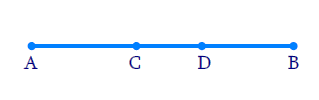# Ex.5.1 Q5 Introduction to Euclids Geometry Solution - NCERT Maths Class 9

Go back to  'Ex.5.1'

## Question

In a Question 4, point $$C$$ is called a mid-point of line segment $$AB$$. Prove that every line segment has one and only one mid-point.

Video Solution
Introduction To Euclids Geometry
Ex 5.1 | Question 5

## Text Solution

Reasoning:

We are aware that the things which coincide with one another are equal to one another.

Steps:

Let us consider that line segment $$AB$$ has two mid points $$‘C’$$ and $$‘D’$$.Let assume $$C$$ is mid-point of $$AB$$

$AC = BC$

Adding $$AC$$ on both sides, we get

\begin{align} AC+AC & = BC+AC \\ 2 AC & = AB \\ AC& = \frac { 1 } { 2 } AB \ldots \ldots ( 1 ) \end{align}

Let us consider a point $$D$$ lying on $$AB,$$ Let assume that $$D$$ be another mid-point of $$AB.$$

Therefore $$AD = BD$$ Adding equal length $$AD$$ on both the sides, we get

\begin{align} AD+AD & = BD+AD \\ 2 AD & = AB \\ AD & = \frac { 1 } { 2 } AB \ldots \ldots ( 2 ) \end{align}

From equations ($$1$$) and ($$2$$), we can conclude that $$AC = AD$$

• $$C$$ Coincides with $$D.$$
• Axiom $$4$$: Things which coincide with one another are equal to one another.
• A line segment has only one midpoint.

Learn from the best math teachers and top your exams

• Live one on one classroom and doubt clearing
• Practice worksheets in and after class for conceptual clarity
• Personalized curriculum to keep up with school